### 靜態函式的兩三事

1. 非靜態函式(也就是類別成員函式)，會依據物件而複製，所以占用記憶體較多

2. 靜態函式的執行速度優於非靜態函式

3. 所以追求效能應該把非靜態改成靜態

4. 那何時該用靜態函式，何時該用非靜態函式，有準則嗎?

這裡我用C# 做為例子，就我記憶所及，Java與C++也是一樣的邏輯，這是物件導向程式語言編譯器的通則，先從非靜態函式是否會依據物件而複製開始談起，驗證的方法很簡單，只要寫一個小類別就可以了。

``````class SimpleClass
{
public int Sum(int x, int y) => x + y;
}

static void Main(string[] args)
{
var c1 = new SimpleClass();
var c2 = new SimpleClass();
c1.Sum(12, 13);
c2.Sum(13, 12);
}``````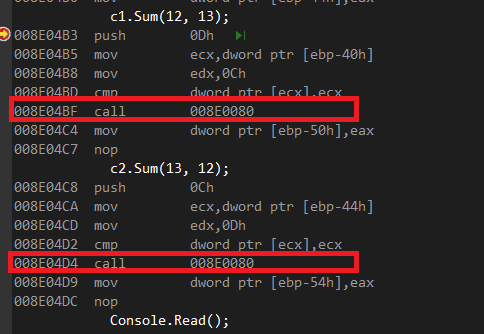Call就是函式呼叫的部分，可以看到是同一個位址，所以這證明了非靜態函式不會依據物件的數量複製。

靜態函式比非靜態函式快，當然，但是差距非常小，這得從函式在編譯器中如何呈現開始說起，靜態函式與非靜態函式在編譯器眼中都是函式(廢話)，差別在於參數數目，也就是this，靜態函式不需要傳入this，而非靜態函式需要傳入this，是的，差距就是一個參數而已，就編譯器角度而言，沒有非靜態函式這種東西，所有的函式都是靜態的，以下面這個例子為例。

``````class Class1
{
public int Sum(int x, int y) => x + y;
public static int Sum2(int x, int y) => x + y;
}

static void Benchmark(Action a, string name)
{
Stopwatch sw = new Stopwatch();
sw.Start();
a();
sw.Stop();
Console.WriteLine(\$"{name} : {sw.ElapsedMilliseconds}");
}

static void Main(string[] args)
{
Benchmark(() =>
{
var c1 = new Class1();
for (int i = 0; i < 1000000000; i++)
c1.Sum(i, i + 2);
}, "instance");
Benchmark(() =>
{
for (int i = 0; i < 1000000000; i++)
Class1.Sum2(i, i + 2);
}, "static");
Benchmark(() =>
{
var c1 = new Class1();
for (int i = 0; i < 1000000000; i++)
c1.Sum(i, i + 2);
}, "instance");
Benchmark(() =>
{
for (int i = 0; i < 1000000000; i++)
Class1.Sum2(i, i + 2);
}, "static");
}``````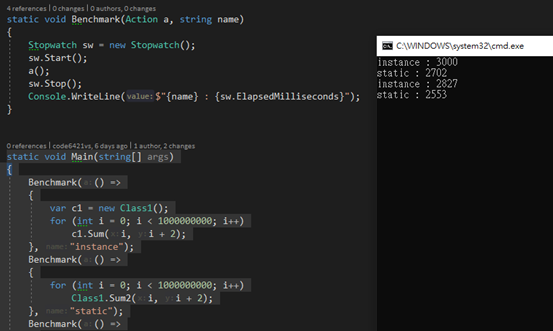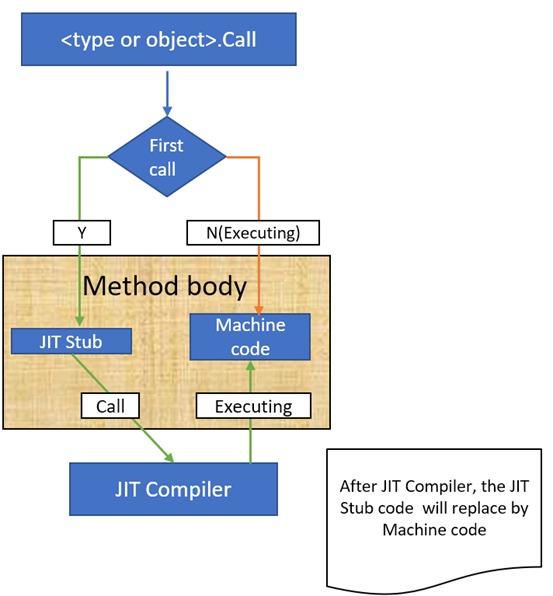``````class Class1
{
public int Sum(int x, int y) => x + y;
public static int Sum2(int x, int y) => x + y;
public static int Sum3(Class1 c, int x, int y) => x + y;
}

static void Benchmark(Action a, string name)
{
Stopwatch sw = new Stopwatch();
sw.Start();
a();
sw.Stop();
Console.WriteLine(\$"{name} : {sw.ElapsedMilliseconds}");
}

static void Main(string[] args)
{
Benchmark(() =>
{
var c1 = new Class1();
for (int i = 0; i < 1000000000; i++)
c1.Sum(i, i + 2);
}, "instance");
Benchmark(() =>
{
for (int i = 0; i < 1000000000; i++)
Class1.Sum2(i, i + 2);
}, "static");
Benchmark(() =>
{
var c1 = new Class1();
for (int i = 0; i < 1000000000; i++)
Class1.Sum3(c1, i, i + 2);
}, "static 2");
Benchmark(() =>
{
var c1 = new Class1();
for (int i = 0; i < 1000000000; i++)
c1.Sum(i, i + 2);
}, "instance");
Benchmark(() =>
{
for (int i = 0; i < 1000000000; i++)
Class1.Sum2(i, i + 2);
}, "static");
Benchmark(() =>
{
var c1 = new Class1();
for (int i = 0; i < 1000000000; i++)
Class1.Sum3(c1, i, i + 2);
}, "static 2");
}``````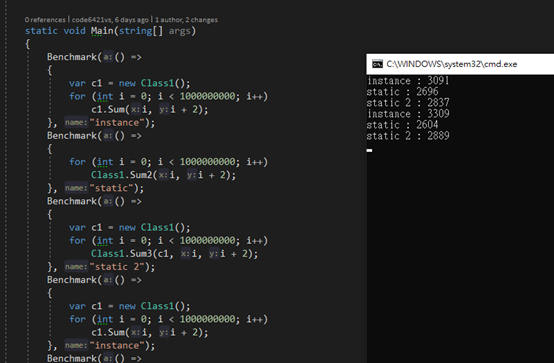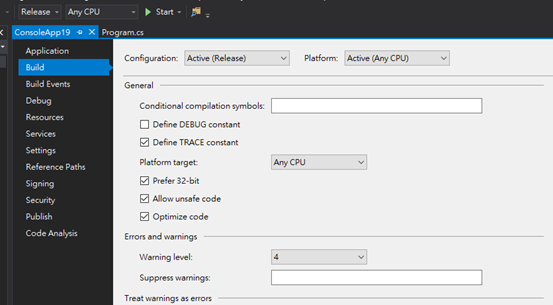``````class Class1
{
[MethodImpl(MethodImplOptions.NoInlining)]
public int Sum(int x, int y) => x + y;
[MethodImpl(MethodImplOptions.NoInlining)]
public static int Sum2(int x, int y) => x + y;
[MethodImpl(MethodImplOptions.NoInlining)]
public static int Sum3(Class1 c, int x, int y) => x + y;
}``````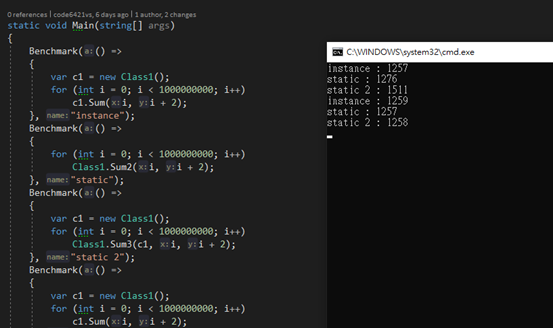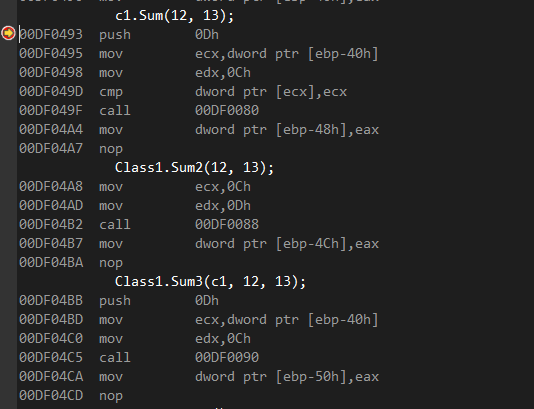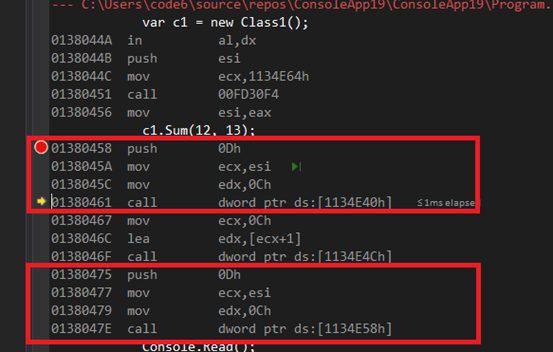除了靜態與非靜態兩種外，在OOP的世界中還有第三種函式，就是可覆載函式，常稱為虛擬函式，這種函式在編譯器的眼裡有不同的呈現方式，以下列程式來說。

``````class Class1

{

[MethodImpl(MethodImplOptions.NoInlining)]

public int Sum(int x, int y) => x + y;

[MethodImpl(MethodImplOptions.NoInlining)]

public static int Sum2(int x, int y) => x + y;

[MethodImpl(MethodImplOptions.NoInlining)]

public static int Sum3(Class1 c, int x, int y) => x + y;

[MethodImpl(MethodImplOptions.NoInlining)]

public virtual int SumVirtual(int x, int y) => x + y;

}

static void Main(string[] args)

{

var c1 = new Class1();

c1.Sum(12, 13);

c1.SumVirtual(12, 13);

Class1.Sum2(12, 13);

Class1.Sum3(c1, 12, 13);

}``````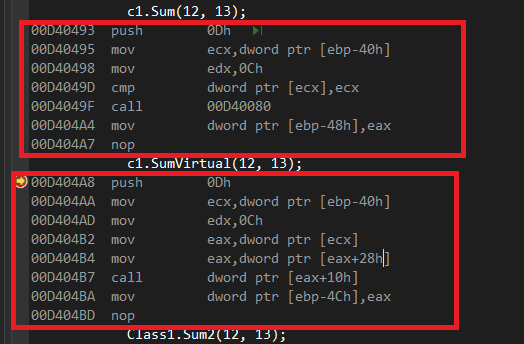靜態函式比非靜態呼叫指令碼有差距，產生效能影響這是事實，但是如果把原本的非靜態改成靜態，必然需要對內容作調整，例如把成員變數改成靜態，或是透過另一個靜態類別來儲存這些變數，這時會帶出兩個問題，一是改成靜態成員變數帶來的混亂，二是改成靜態類別又落入了間接存取的狀況，這通常會把省下的那一個指令時間加回來，正負得零，只是徒工。靜態與非靜態應該回歸設計，函式會設計成靜態通常是因為其沒有狀態，沒有side effect，這在.NET Framework中有很多例子可以參考，例如Math.Abs為何是靜態？因為他沒有狀態，同值呼叫會得到同樣的結果，所以沒有Side Effect，做為靜態很合理。String.ToString()為何是非靜態，因為她需要狀態，如果設計成靜態就變成了String.ToString(myString)，這時靜態與非靜態就會等值(排除ToString其實是虛擬函式的這件事)。